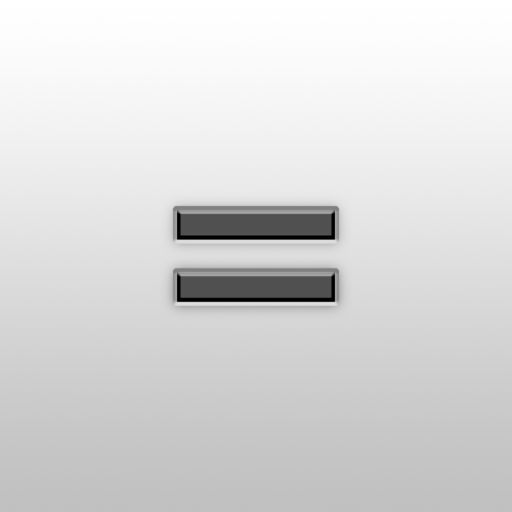Calc Table# Calc Table

## Kenjirou Uesaka

1.0.2 for iPhone
Free
0

0 Ratings

Release Date

2017-10-19

Size

41.3 MB

### Screenshots for iPhone

iPhone
Calc Table Description
It is a new sense calculator that can arrange calculators and memos with basic functions as much as you want.
Of course you can use all functions free of charge.

Create a calculator, give it a name, move freely over the field,
It also has a function to compute selected calculators collectively.

It is perfect for you to calculate simple calculator calculator app.

· Basic functions of the calculator
Arithmetic operation (addition, subtraction, multiplication, division)
Percentage calculation
Decimal calculation
Back key that can be traced back to the formula
By pressing equals multiple times at the time of calculation, the previous calculation is performed

· Useful functions
Placing notes
Move calculator or memo
Select range to select calculator and memo at the same time
Calculate the selected calculator at once (long press the calculator addition button)
Calculate calculator and calculator
▼ Compact Calculator Size with Button
Even if you close the application, the calculation result remains intact
Calc Table 1.0.2 Update
2017-10-19 Version History
Fixed bug that sound effect is not playing.
More
Price:
Free
Version:
1.0.2
Size:
41.3 MB
Release Date:
2017-10-19
Developer:
Kenjirou Uesaka
Language:
English
More
You May Also Like
Developer Apps